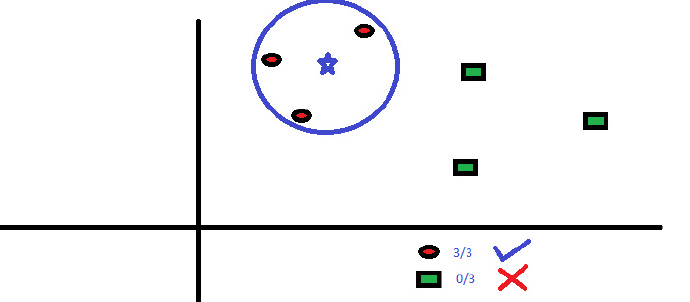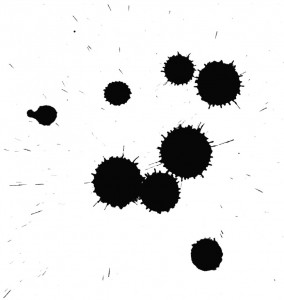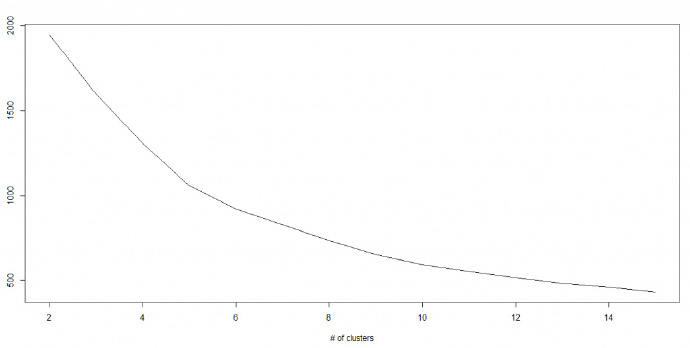﻿ [转]10种机器学习算法Part.2-FunnyWeb

# [转]10种机器学习算法Part.2

2016-10-17 01:02:21905 views

## 点击跳转至本文Part1

http://www.analyticsvidhya.com/blog/2015/08/common-machine-learning-algorithms/

## 6、KNN（K – 最近邻算法）• KNN 的计算成本很高。

• 变量应该先标准化（normalized），不然会被更高范围的变量偏倚。

• 在使用KNN之前，要在野值去除和噪音去除等前期处理多花功夫。

#### Python Code

`#Import Libraryfrom sklearn.neighbors import KNeighborsClassifier#Assumed you have, X (predictor) and Y (target) for training data set and x_test(predictor) of test_dataset# Create KNeighbors classifier object model KNeighborsClassifier(n_neighbors=6) # default value for n_neighbors is 5# Train the model using the training sets and check scoremodel.fit(X, y)#Predict Outputpredicted= model.predict(x_test)`

R Code

`library(knn)x <- cbind(x_train,y_train)# Fitting modelfit <-knn(y_train ~ ., data = x,k=5)summary(fit)#Predict Output predicted= predict(fit,x_test)`

## 7、K 均值算法 K-Means

K – 均值算法是一种非监督式学习算法，它能解决聚类问题。使用 K – 均值算法来将一个数据归入一定数量的集群（假设有 k 个集群）的过程是简单的。一个集群内的数据点是均匀齐次的，并且异于别的集群。K – 均值算法怎样形成集群：

1. K – 均值算法给每个集群选择k个点。这些点称作为质心。

2. 每一个数据点与距离最近的质心形成一个集群，也就是 k 个集群。

3. 根据现有的类别成员，找出每个类别的质心。现在我们有了新质心。

4. 当我们有新质心后，重复步骤 2 和步骤 3。找到距离每个数据点最近的质心，并与新的k集群联系起来。重复这个过程，直到数据都收敛了，也就是当质心不再改变。

K – 均值算法涉及到集群，每个集群有自己的质心。一个集群内的质心和各数据点之间距离的平方和形成了这个集群的平方值之和。同时，当所有集群的平方值之和加起来的时候，就组成了集群方案的平方值之和。#### Python Code

`#Import Libraryfrom sklearn.cluster import KMeans#Assumed you have, X (attributes) for training data set and x_test(attributes) of test_dataset# Create KNeighbors classifier object model k_means = KMeans(n_clusters=3, random_state=0)# Train the model using the training sets and check scoremodel.fit(X)#Predict Outputpredicted= model.predict(x_test)`

R Code

`library(cluster)fit <- kmeans(X, 3) # 5 cluster solution`

## 8、随机森林 Random Forest

1. 如果训练集的案例数是 N，则从 N 个案例中用重置抽样法随机抽取样本。这个样本将作为“养育”树的训练集。

2. 假如有 M 个输入变量，则定义一个数字 m<<M。m 表示，从 M 中随机选中 m 个变量，这 m 个变量中最好的切分会被用来切分该节点。在种植森林的过程中，m 的值保持不变。

3. 尽可能大地种植每一棵树，全程不剪枝。

Python

`#Import Libraryfrom sklearn.ensemble import RandomForestClassifier#Assumed you have, X (predictor) and Y (target) for training data set and x_test(predictor) of test_dataset# Create Random Forest objectmodel= RandomForestClassifier()# Train the model using the training sets and check scoremodel.fit(X, y)#Predict Outputpredicted= model.predict(x_test)`

R Code

`library(randomForest)x <- cbind(x_train,y_train)# Fitting modelfit <- randomForest(Species ~ ., x,ntree=500)summary(fit)#Predict Output predicted= predict(fit,x_test)`

## 9、降维算法  Dimensionality Reduction Algorithms

#### Python  Code

`#Import Libraryfrom sklearn import decomposition#Assumed you have training and test data set as train and test# Create PCA obeject pca= decomposition.PCA(n_components=k) #default value of k =min(n_sample, n_features)# For Factor analysis#fa= decomposition.FactorAnalysis()# Reduced the dimension of training dataset using PCAtrain_reduced = pca.fit_transform(train)#Reduced the dimension of test datasettest_reduced = pca.transform(test)#For more detail on this, please refer  this link.`

#### R Code

`library(stats)pca <- princomp(train, cor = TRUE)train_reduced  <- predict(pca,train)test_reduced  <- predict(pca,test)`

`#Import Libraryfrom sklearn.ensemble import GradientBoostingClassifier#Assumed you have, X (predictor) and Y (target) for training data set and x_test(predictor) of test_dataset# Create Gradient Boosting Classifier objectmodel= GradientBoostingClassifier(n_estimators=100, learning_rate=1.0, max_depth=1, random_state=0)# Train the model using the training sets and check scoremodel.fit(X, y)#Predict Outputpredicted= model.predict(x_test)`
`library(caret)x <- cbind(x_train,y_train)# Fitting modelfitControl <- trainControl( method = "repeatedcv", number = 4, repeats = 4)fit <- train(y ~ ., data = x, method = "gbm", trControl = fitControl,verbose = FALSE)predicted= predict(fit,x_test,type= "prob")[,2]`